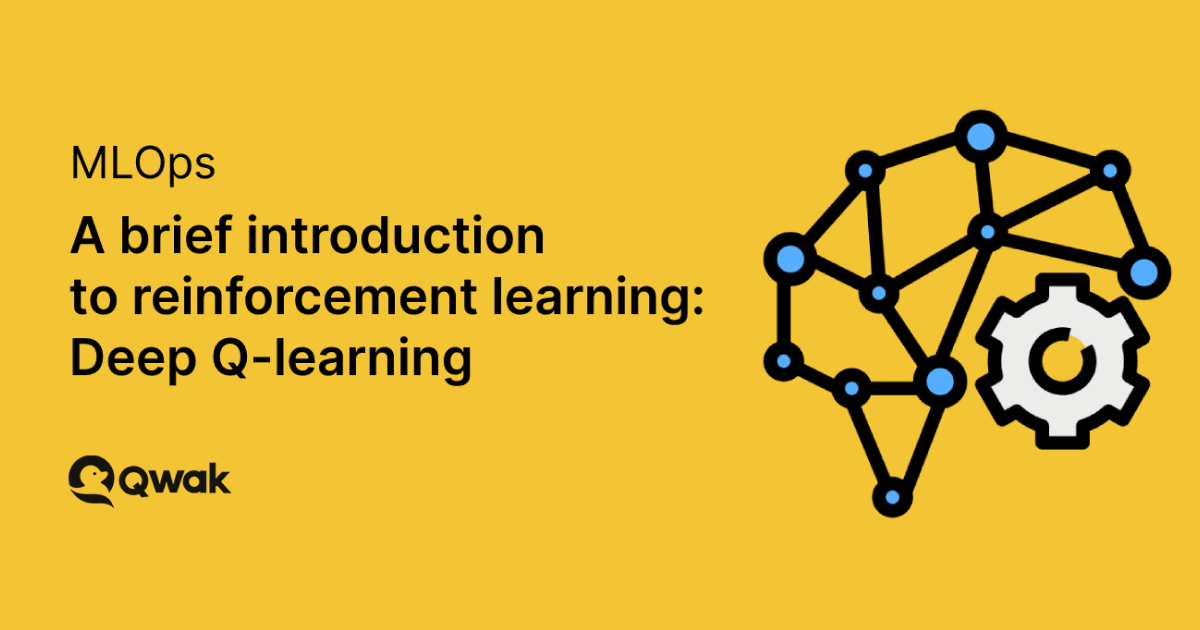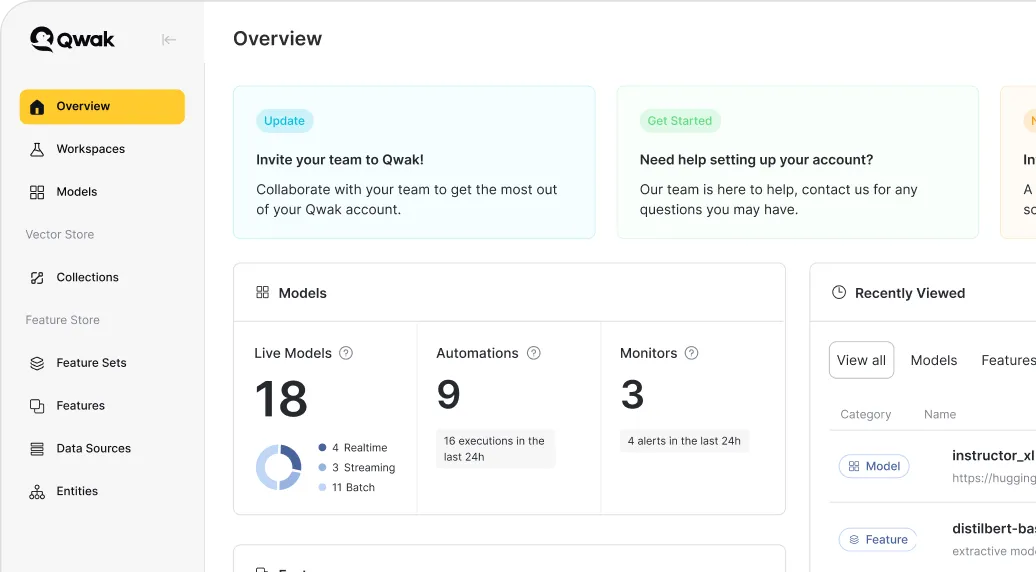# A brief introduction to reinforcement learning: Deep Q-learning

In our previous reinforcement learning blog post, we explored why reinforcement learning is an exciting field in AI and machine learning.Alon Lev
Co-Founder & CEO at Qwak
May 27, 2022In our previous reinforcement learning blog post, we explored why reinforcement learning is an exciting field in AI and machine learning. One of the main reasons for this is due to major breakthroughs that have enabled computer programs such as Alpha Go to achieve human-level performance playing games such as ‘Go’, even beating reigning world champions.

As we learned, one of the core concepts in reinforcement learning is the Q-learning algorithm, and in this blog post we’re going to dive deeper into the workings of it and look at how it can be taken one step further with deep Q-learning.

But first, let’s quickly recap.

## What is reinforcement learning?

In short, reinforcement learning is a field of machine learning that involves training machine learning models to make a sequence of desired decisions and/or carry out the desired action.

In reinforcement learning, the model learns to achieve a goal in an uncertain and potentially complex environment, typically through a game-like situation, using trial and error to come up with a solution to the problem it is faced with.

For example, if you want to teach a dog to sit down by bribing it with treats, it won’t understand you at first. It might respond to your command of “Sit down!” by carrying out random actions. At some point, however, it will sit down, and it will be rewarded with a treat. When this scenario is iterated enough times, the dog will figure out that to receive a treat, it needs to sit down when it hears your verbal cue.

## Q-learning

Machine learning teams can use a variety of methods and algorithms to teach their models. One of the most popular is Q-learning, which is a value-based reinforcement learning algorithm used to find the optimal action-selection policy by using a Q function.

We covered this in depth in our previous blog post — A brief introduction to reinforcement learning: Q-learning — and we suggest reading this first.

Let’s look at Q-learning in more depth by using the CartPole environment as an example.

In the CartPole environment, the objective is to move a cart left or right to balance an upright pole. The state space is described with four values: Cart Position, Cart Velocity, pole Angle, and Pole Velocity, while the action space is described with two values, zero or one. This allows the cart to either move left or right at each step.

In ‘normal’ (non-deep) Q-learning, we would first initialize our Q-table as described in the previous article, choose an action using the Epsilon-Greedy Exploration Strategy, and then update the Q-table using the Bellman Equation

### Initializing the Q-table

As discussed previously, the Q-table is a data structure that is used to track the states, actions, and their expected rewards. More specifically, the Q-table maps a state-action pair to a Q-value (the estimated optimal future value) which the agent (model) will learn.

Here’s an example of a Q-table:

State-ActionQ-Value
(S1, A1)0
(S0, A3)0
(S2, A2)0

At the start of the Q-learning process, all Q-table values are zero. As the agent carries out different actions through trial and error, it learns each state-action pair’s expected reward and updates the table with the new Q-value (exploration).

The Q-learning algorithm’s goal is to learn the q-value for a new environment, which is the maximum expected reward an agent can receive by carrying out an action (a) from the state (s). Once the agent has learned the Q-value of each state-action pair, the agent at state (s) maximizes its reward by choosing the action (a) with the highest expected reward (exploitation).

### Choosing an action using the Epsilon-Greedy Exploration Strategy

The Epsilon-Greedy Exploration Strategy is a common method for tackling the exploration-exploitation. It works as follows:

1. When it’s time to choose an action, roll a die
2. If the die has a probability less than epsilon, choose a random action
3. Else, take the best known action at the agent’s current state

Keep in mind that in the beginning, every step that the agent takes will be random, which is useful to enable the agent to learn about its environment. As it takes more and more steps, however, the value of epsilon diminishes, and the agent will try to take the optimum actions it has learned. Towards the end of the training process, the agent will be exploring less and exploiting more.

### Updating the Q-table using the Bellman Equation

The Bellman Equation shows us how we can update the Q-table after each step. We explored this in the previous article, so to summarize, the agent updates the current perceived value with the estimated future reward. In deployment, the agent will search through all actions for a particular state and choose the best state-action pair, i.e., the one with the highest Q-value.

In this equation:

• S is the State or observation
• A is the Action the agent takes
• R is the Reward from taking an Action
• t is the time step
• α is the Learning Rate
• λ is the discount factor which causes rewards to lose their value over time so that more immediate rewards are prioritized

After several iterations, the Q-table will be populated with values, for example:

State-ActionQ-Value
(S1, A1)9
(S0, A3)4
(S2, A2)1

## Deep Q-learning

While regular Q-learning maps each state-action pair to its corresponding value, deep Q-learning uses a neural network to map input states to pairs via a three-step process:

• Initializing Target and Main neural networks
• Choosing an action
• Updating network weights using the Bellman Equation

### Initializing Target and Main neural networks

The main difference between deep and regular Q-learning is the implementation of the Q-table. In deep Q-learning, this is replaced with two neural networks that handle the learning process.

While these networks have the same overarching architectures, they have different weights. Every N steps, the weights from the Main network are copied to the Target network. Using both networks helps to stabilize the learning process so that the algorithm can learn more effectively. In our example implementation below, the Main network weights replace the Target network weights after every 60 steps.

The Main and Target neural networks map input states to a pair. In this case, each output node (A, which represents an action) contains its Q-value as a floating point number. In the above example, one output has a Q-value of eight while the other has a value of five.

### Choosing an action using the Epsilon-Greedy Exploration Strategy

In the Epsilon-Greedy Exploration strategy, the agent chooses a random action with probability epsilon and exploits the best known action at that state. This is always going to be the action that has the largest predicted Q-value.

### Updating the Q-table using the Bellman Equation

After the agent chooses an action, it performs it before updating the Main and Target networks according to the Bellman equation. Deep Q-learning agents use a process known as experience reply—the act of storing and replaying game states that the reinforcement learning algorithm can learn from—to learn about their environments and subsequently update the Main and Target networks.

The Main network samples and trains on a batch of past experiences every four steps. These weights are then copied to the Target network every 60 steps. Just like in regular Q-learning, however, the agent must still update model weights according to the Bellman Equation.

The old Q-value of 8 is replaced with the new value of 9, meaning the network can be re-trained. From the original Bellman Equation above, we need to replicate the temporal difference target operation using the neural network rather than the Q-table.

Keep in mind that the Target network, not the Main network, is used to calculate the temporal difference target. If the temporal difference target operation produces a value of 9, the Main network weights can be updated by assigning 9 to the target Q-value and fitting the Main network weights to the new target values.

## Remember, this is a brief introduction

Training a very, very simple simulation like this by using a deep neural network isn’t exactly optimal. First, the simulation isn’t exactly nuanced, and deep networks thrive in more complex scenarios. Notably, challenging aspects of operationalizing a model is integrating the different phases of the model lifecycle, from generating features from raw data, training the model, and making predictions. These phases become more complex in large organizations that span to different teams.

In such a case, the feature store creates a collaboration platform where teams create features for model training. However, keep in mind that this is a brief introduction and further research on this topic is encouraged.

That aside, now that you have a basic understanding of the difference between basic Q-learning and deep Q-learning, including the fundamentals of replicating a Q-table with a neural network, you can have a go of tackling more complicated simulations by using the Qwak platform.

At Qwak, we help businesses unify their ML engineering and data operations, providing agile infrastructure that enables the continuous productionization of ML models at scale. If you’re interested in learning more, check out our platform here.

Chat with us to see the platform live and discover how we can help simplify your ML journey.